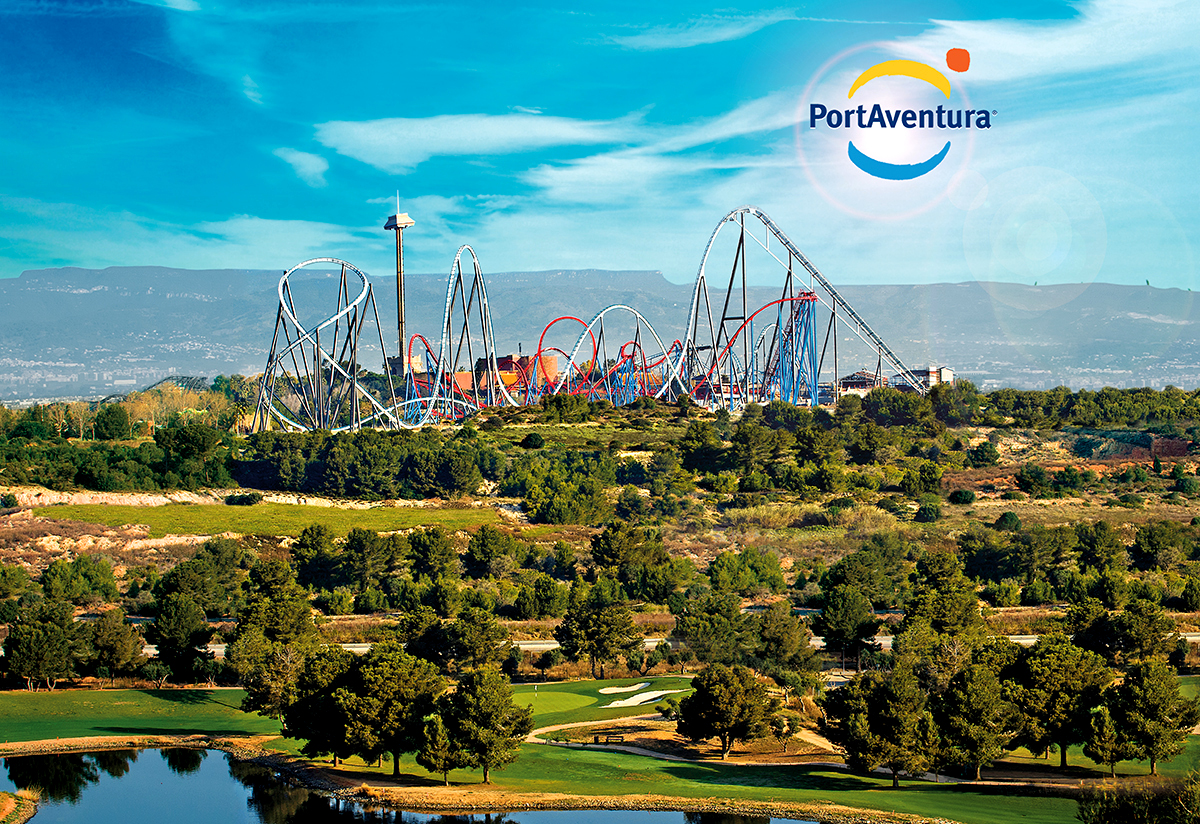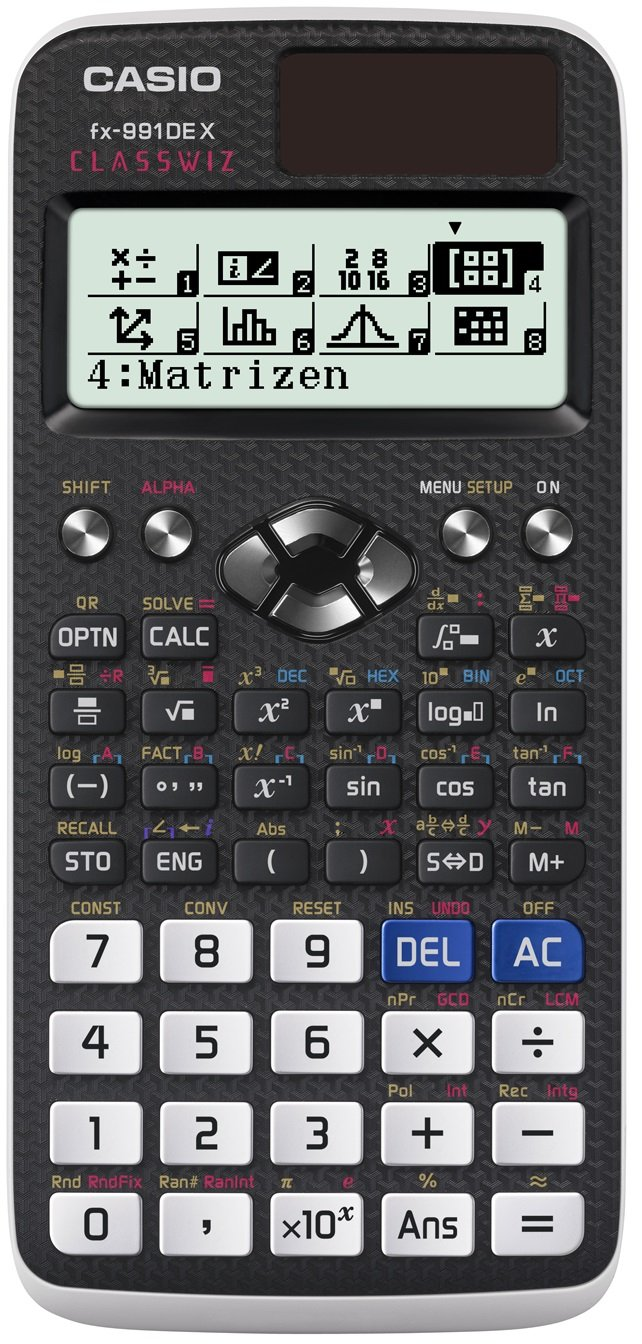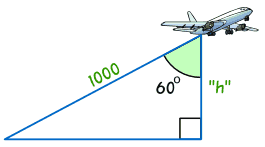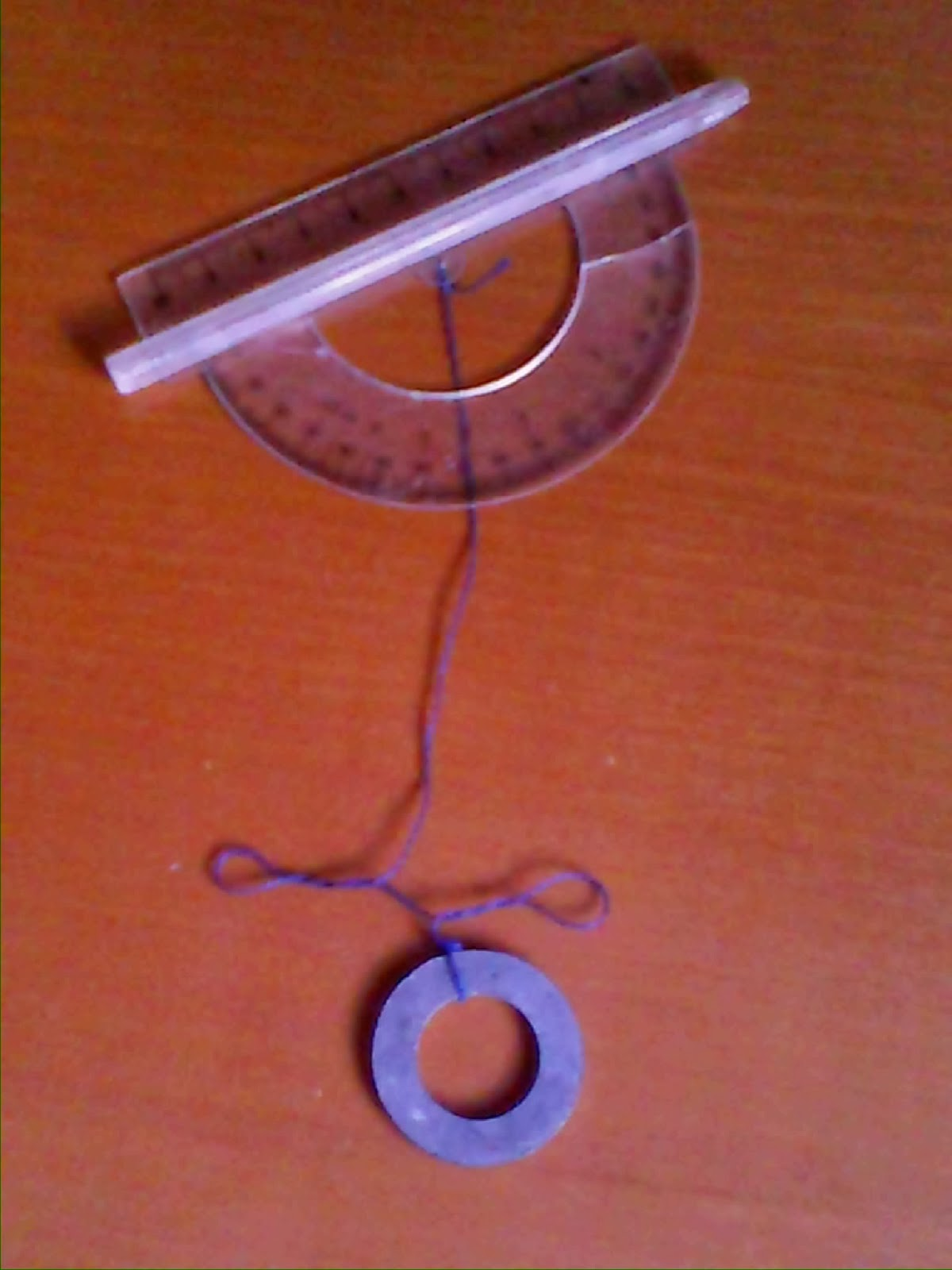This is a Clilstore unit. You can .

# Science and culture in amusement parks

Amusement parks are places where Science and Maths work together. In this unit we will learn Maths and Physics contents applied in Port Aventura.LESSON 1: TRIGONOMETRY

SESSION 2: Trigonometric ratios

Review of previous conceps (Thales and Pythagoras theorems)

Exercise 1:HURAKAN CONDOR

Javi is 1.80m tall, and his shadow is 0.74m long. At the same time, the shadow of Hurakan Condor is 47m long. Which is the height of this free fall ride?

Exercise 2:TUTUKI SPLASH

The ride named Tutuki Splash finishes with a fall 17m long. If the displacement is 6m, which is the height of the ride?

Instruction

Watch this video and write on your notebook how to calculate the trigonometric ratios of a right angled triangle (sine, cosine, tangent)

Trigonometric ratios

(Remember that you can choose watching the video with subtitles)

Activities

1. Calculate the trigonometric ratios of the following angles A and C:2. Calculate the trigonometric ratios of the angles of the fall of tutuki Splash.

SESSION 3: Find the angle

Exercise: Calculate the trigonometric ratios of A and BInstructionHow to use your calculator:If you know the sine, the cosine or the tangent of an angle, you can use your calculator in order to find the value of the angle. Use the key "SHIFT" and "sin-1 " or "arcsin" (depending on the model of calculator) and the value of the sine (for example, 0.5) Then you will get the angle whose sine is 0.5. The same happens with arcos or arctan. Be carefull, you have to set up degrees as angle unit on your calculator.

Exercises:

1. Find the angles using your calculator:

sin A=0,66    A=

cos B= 0,5    B=

tan C=1         C=

2. Solve the triangle (find the 3 sides and the 3 angles):SESSION 4: Calculate heights

Exercise: Solve the following triangle (1-2-4)Instruction: 2 solved exercises

Exercises:

1. Calculate the height "h" (1-2-4)2. Mary sees a tree with an angle of 40º, and if she goes back 4m, the angle is 28º. Which is the height of the tree? (pencils at the center)

SESSION 5: THE GONIOMETER

Goniometer: materials: protactor, thread, a nut, adhesive tape, a strawWhen you look at an object with you goniometer, you get an angle, which is the same as the angle that your eyesight does with the height of the object.

Practise:

With your goniometer and your metre rule, find the height of some buildings near you.

angle 1:

distance gone back:

angle 2:

Calculations:

LESSON 2: ENERGY

SESSION 1

Review:

Watch the following video and comment what happens.

The ball and the feather

Exercise 1:

The ride Furius Baco changes its velocity from 0 to 135 km/h in 3 seconds, calculate its acceleration.Exercise 2: An apple falls from the top of a tree. If the tree is 5m tall, calculate:

a) the time that the apple needs to get to the ground.

b) final velocity when the apple arrives the ground

SESSION 2 and 3

Instruction

- Kinetic and potential energy  (from minute 0 to 9.37)

- Pendulum: https://www.youtube.com/watch?v=xXXF2C-vrQE

Solved problem

Exercise 2: You calculated the height of Hurakan Condor in Session 1 of the first lesson, now watch the following video and time the free fall. With this information, calculate the maximum velocity and the acceleration of the ride.

Hurakan Condor

Exercise 3: with the information in this web: https://en.wikipedia.org/wiki/Dragon_Khan and pictures on the internet, do a map of Dragon Khan (including the loops) and find the velocity at 5 different points of the ride.

SESSION 4 AND 5

Visit the following webs and then explain them to your teammates

Finally, do a digital presentation (PPT, Prezi....)

Short url:   http://multidict.net/cs/7393